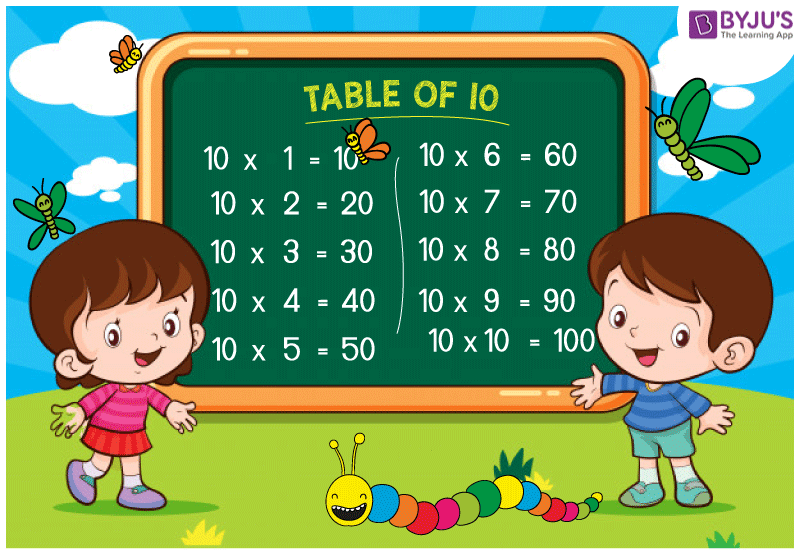# Table of 10

Table of 10 is provided here so that students can memorize and perform fast calculations. Doing fast calculation will help students to save a lot of time in the examination, where the number of questions is more as compared to the time allotted. To help students, the 10 × 20 table is provided here along with the 10 times table pdf which can be referred in offline mode also.## Table of 10

 10 × 1 = 10 10 × 2 = 20 10 × 3 = 30 10 × 4 = 40 10 × 5 = 50 10 × 6 = 60 10 × 7 = 70 10 × 8 = 80 10 × 9 = 90 10 × 10 = 100 10 × 11 = 110 10 × 12 = 120 10 × 13 = 130 10 × 14 = 140 10 × 15 = 150 10 × 16 = 160 10 × 17 = 170 10 × 18 = 180 10 × 19 = 190 10 × 20 = 200

Visit BYJU’S for  latest CBSE study materials and syllabus. We also provide subject wise study CBSE notes for 6 – 12.